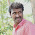## Saturday, January 21, 2012

### Send Color Text In Face Book Chat

Hi  Friends if you want to send color text in Facebook ,Just Copy the following codes and past the code in your Facebook chat box. Please give space between each letter or code..

Codes For Letters:

[] = A
[] = B
[] = C
[] = D
[] = E
[] = F
[] = G
[] = H
[] = I
[] = J
[] = K
[] = L
[] = M
[] = N
[] = O
[] = P
[] = Q
[] = R
[] = S
[] = T
[] = U
[] = V
[] = W
[] = X
[] = Y & [] = Z

#### 1 comment:

1.